# Two Sample t Test with Minitab

### What is Two Sample T Test?

Two sample t test is a hypothesis test to study whether there is a statistically significant difference between the means of two populations.

• Null Hypothesis (H0): μ1 = μ2
• Alternative Hypothesis Ha) : μ1μ2

Where: μ1 is the mean of one population and μ2 is the mean of the other population of our interest.

#### Assumptions of Two Sample T Tests

• The sample data drawn from both populations are unbiased and representative.
• The data of both populations are continuous.
• The data of both populations are normally distributed.
• The variances of both populations are unknown.
• Two sample t-test is more robust than a z-test when the sample size is small (< 30).

#### Three Types of Two Sample T Tests

1. Two sample t test when the variances of two populations are unknown but equal
Two sample t test (when σ21 = σ22)
2. Two sample t test when the variances of the two population are unknown and unequal
Two sample t test (when σ21 ≠ σ22)
3. Paired t test when the two populations are dependent of each other, so each data point from one distribution corresponds to a data point in the other distribution.

### Test of Equal Variance

To check whether the variances of two populations of interest are statistically significant different, we use the test of equal variance.

• Null Hypothesis (H0): σ21 = σ22
• Alternative Hypothesis (Ha): σ21 ≠ σ22

An F-test is used to test the equality of variances between two normally distributed populations.
An F-test is a statistic hypothesis test in which the test statistic follows an F-distribution when the null hypothesis is true. The most known F-test is the test of equal variance for two normally distributed populations. The F-test is very sensitive to non-normality. When any one of the two populations is not normal, we use the Brown–Forsythe test for checking the equality of variances.

### Decision Rules of a Two Sample T Test

• Null Hypothesis (H0): μ1 = μ2
• Alternative Hypothesis(Ha) : μ1μ2

If |tcalc| > tcrit, we reject the null and claim there is a statistically significant difference between the means of the two populations.
If |tcalc| < tcrit, we fail to reject the null and claim there is not any statistically significant difference between the means of the two populations.

### Use Minitab to Run a Two-Sample T Test

Case study: We are trying to compare the average retail price of a product in state A and state B.

Data File: “Two-Sample T Test” tab in “Sample Data.xlsx”• Null Hypothesis (H0): μ1 = μ2
• Alternative Hypothesis(Ha) : μ1μ2

In this example we will be comparing the average price of a product in two different states. The null hypothesis is that the price in state A is equal to the price in state B.

Step 1: Test the normality of the retail price for both state A and B.

1. Click Stat → Basic Statistics → Graphical Summary.
2. A window named “Graphical Summary” pops up.
3. Select “Retail Price” as the “Variables.”
4. Click in the blank box right below “By variables (optional)” and “State” appears in the list box on the left.
5. Select the “State” as the “By variables (optional).”6. Click “OK.”
7. The normality test results would appear in the new windows.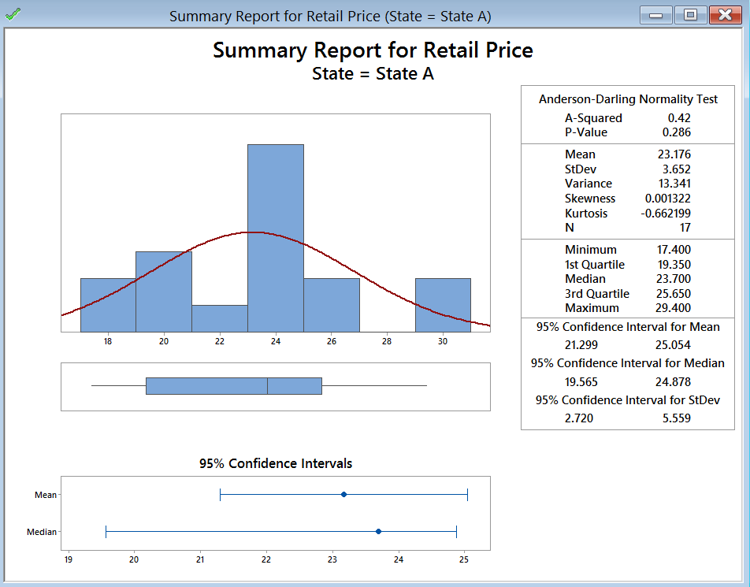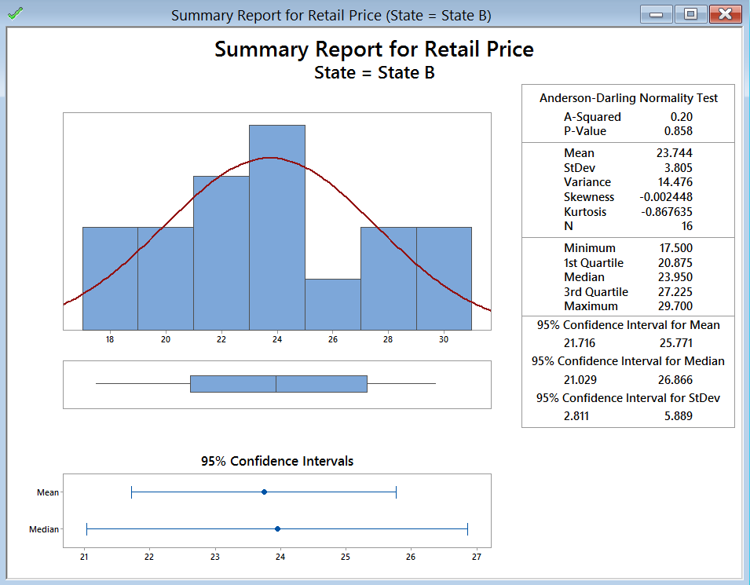• Null Hypothesis(H0): The data are normally distributed.
• Alternative Hypothesis(Ha): The data are not normally distributed.

Both retail price data of state A and B are normally distributed since the p-values are both greater than alpha level (0.05). If any of the data series is not normally distributed, we need to use other hypothesis testing methods other than the two-sample t-test.

By following the instructions on the previous page, we first determine if the data follow a normal distribution. In this case, you can see that the p-value for both is higher than 0.05, so we fail to reject the null hypothesis that the data are normally distributed. If the data are not normally distributed, we must use a different test.

Step 2: Test whether the variances of the two data sets are equal.

• Null Hypothesis(H0):
• Alternative Hypothesis(Ha):
1. Click Basic Statistics → 2 Variances.
2. A new window named “Two-SampleVariance” pops up.
3. Click in the blank box of “Samples” and “Retail Price” appears in the list box on the left.
4. Select “Retail Price” as the “Samples.”
5. Click in the blank box of “SampleIDs” and “State” appears in the list box on the left.
6. Select “State” as the “Sample”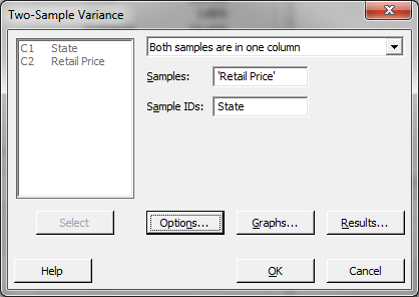7. Click “Options” and select
“Use test and confidence intervals based on normal distribution”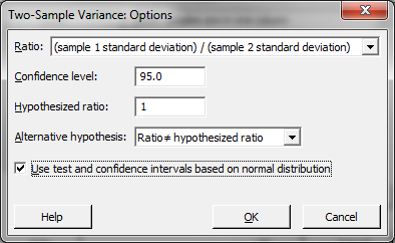8. Click “OK.” in the “Options” window.
9. Click “OK” in the 2-Samplet window
10. The results show up in the session window.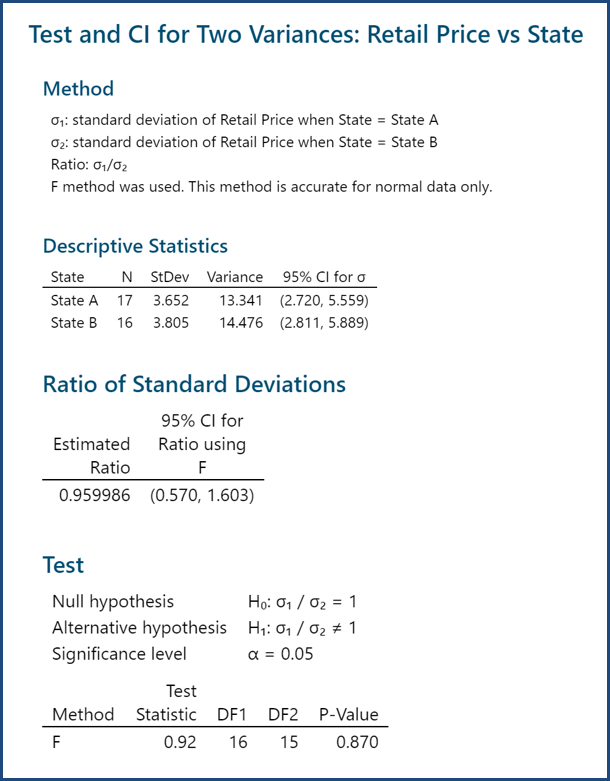Because the retail prices at state A and state B are both normally distributed, an F test is used to test their variance equality. The p-value of F test is 0.870, greater than the alpha level (0.05), so we fail to reject the null hypothesis and we claim that the variances of the two data sets are equal. We will use the two-sample t-test (when σ21 = σ22) to compare the means of the two groups. If σ21 ≠ σ22, we will use the two-sample t-test (when σ21σ22) to compare the means of the two groups.

Step 3: Run two-sample t-test to compare the means of two groups.

1. Click Stat → Basic Statistics → 2-Sample
2. A new window named “Two-Samplet for the Mean” pops up.
3. Click in the blank box right next to “Samples” and the “Retail Price” appears in the list box on the left.
4. Select “Retail Price” as the “Samples.”
5. Click in the blank box right next to “SampleIDs” and the “State” appears in the list box on the left.
6. Select “State” as the “Subscripts.”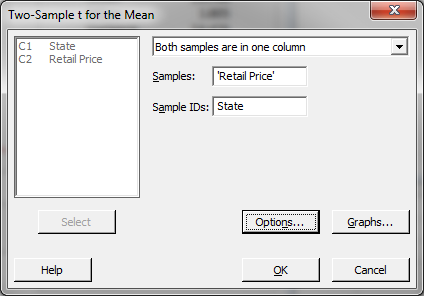7. Click “Options”
8. Check the box “Assume equal variances.”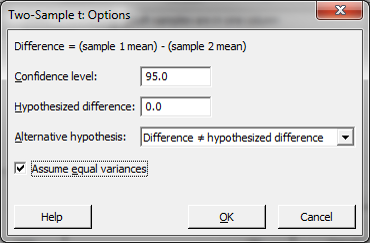9. Click “OK.” in the “Options” window.
10. Click “OK” in the 2-Samplet window
The results of the two-sample t-test (when σ1 = σ2) appear in the session window.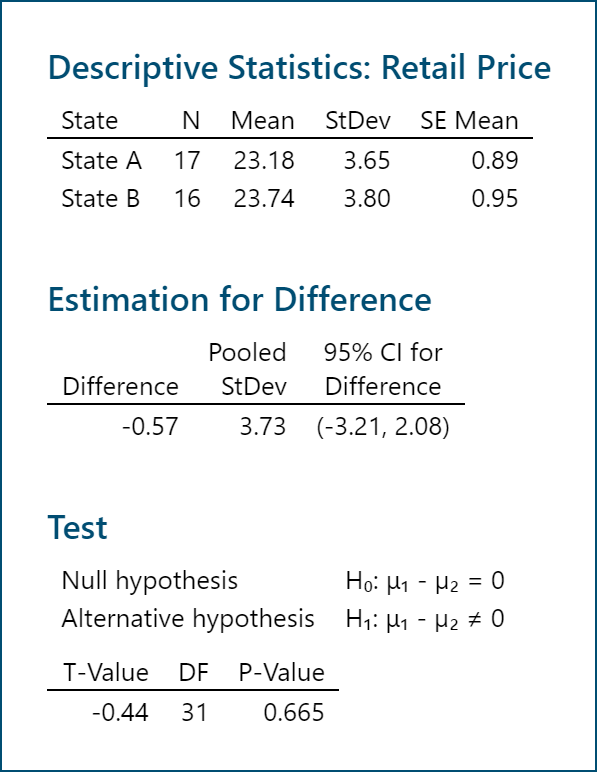Since the p-value of the t-test (assuming equal variance) is 0.665, greater than the alpha level (0.05), we fail to reject the null hypothesis and we claim that the means of the two data sets are equal. If the variances of the two groups do not equal, we will need to use the two-sample t-test (when σ1σ2) to compare the means of the two groups. In the window of “2-Sample t: Options)”, uncheck the box next to “Assume equal variances” and run the 2-sample t-test again.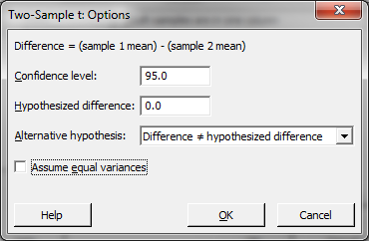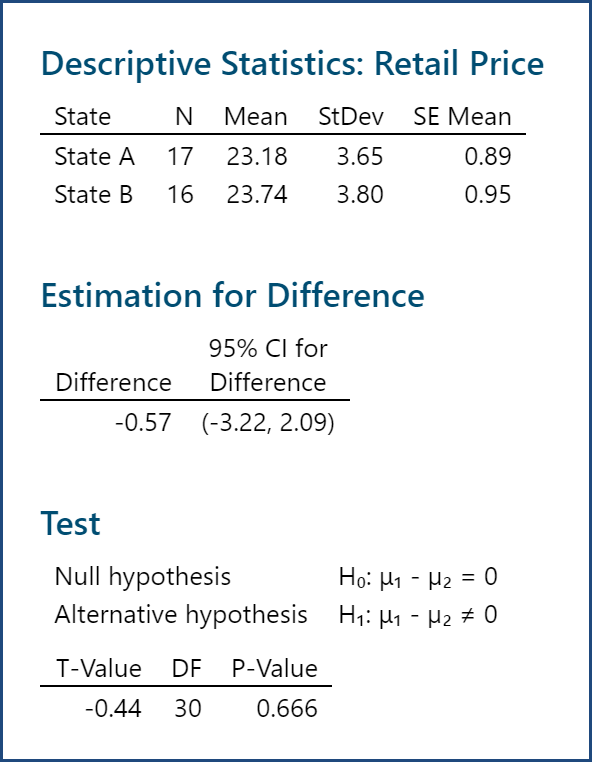Model summary: Since the p-value of the t-test (assuming unequal variance) is 0.666, greater than the alpha level (0.05), we fail to reject the null hypothesis and we claim that the means of two groups are equal.